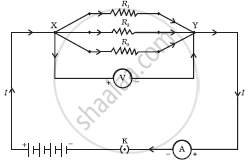Share

# Resistance of a System of Resistors - Resistors in Parallel

Course

#### description

• parallel combination of resistors and its applications in daily life

#### notes

Resistors in parallel:When resistors are joined in parallel, the reciprocal of total resistance of the system is equal to the sum of reciprocal of the resistance of resistors.

If total resistance = R
Resistance of resistors are R1,R2,R3,.....Rn.

Therefore R= 1/("R"1)+1/("R"2)+1/("R"3).....1/("Rn")

In parallel the Current (I) varies and voltage (V) remains the same.

### Shaalaa.com

Electricity part 8 (Resistor Parallel & series) [00:11:32]
S
series 1
0%

S